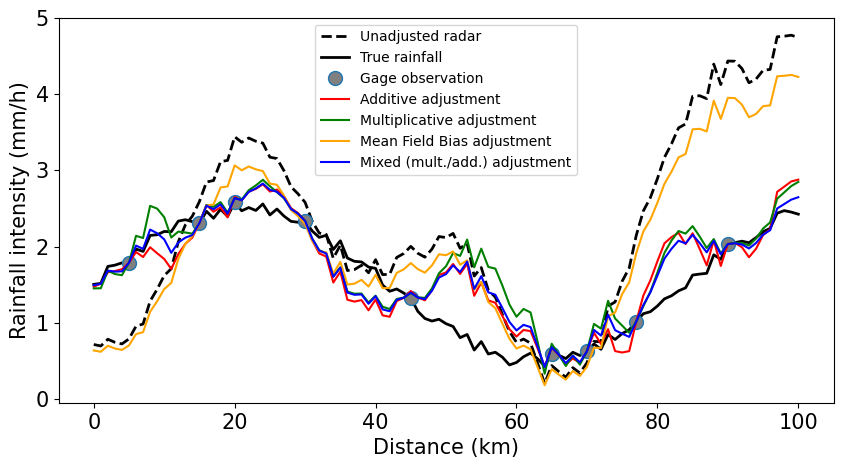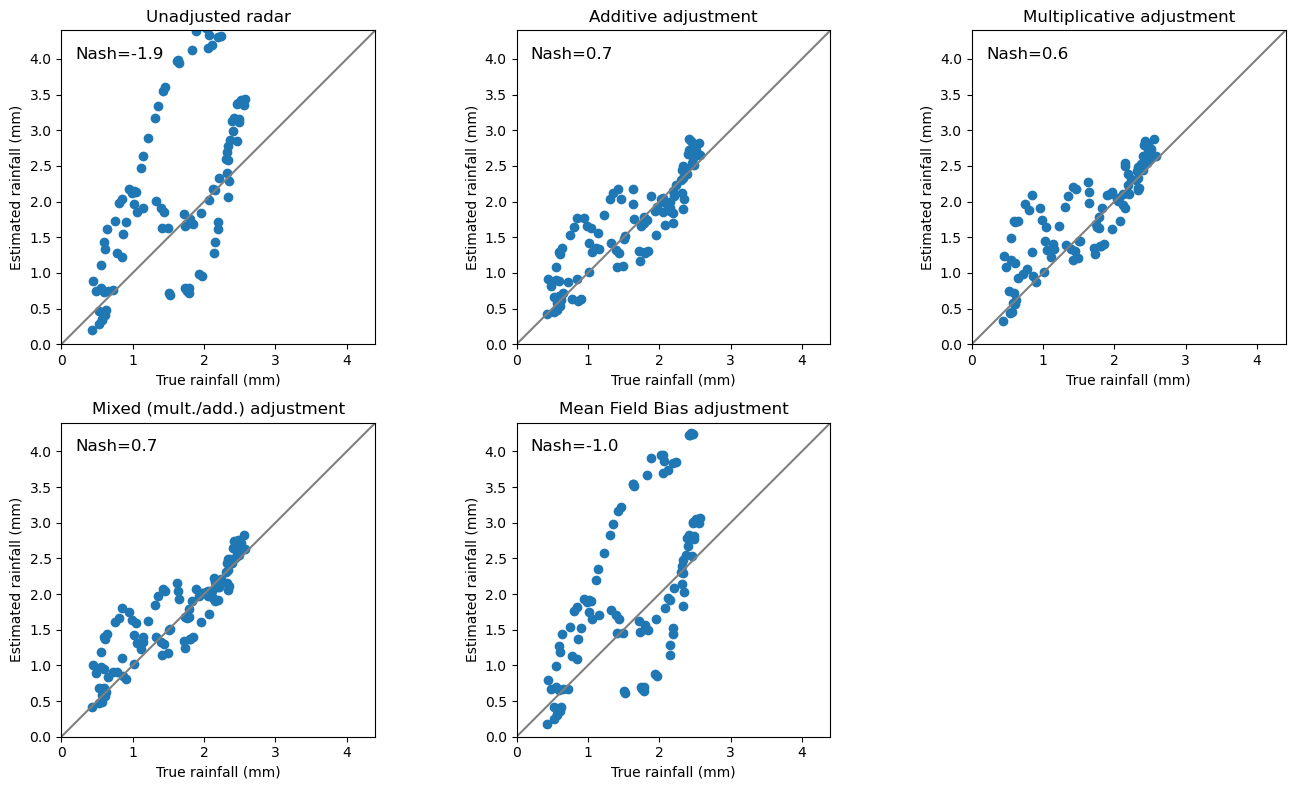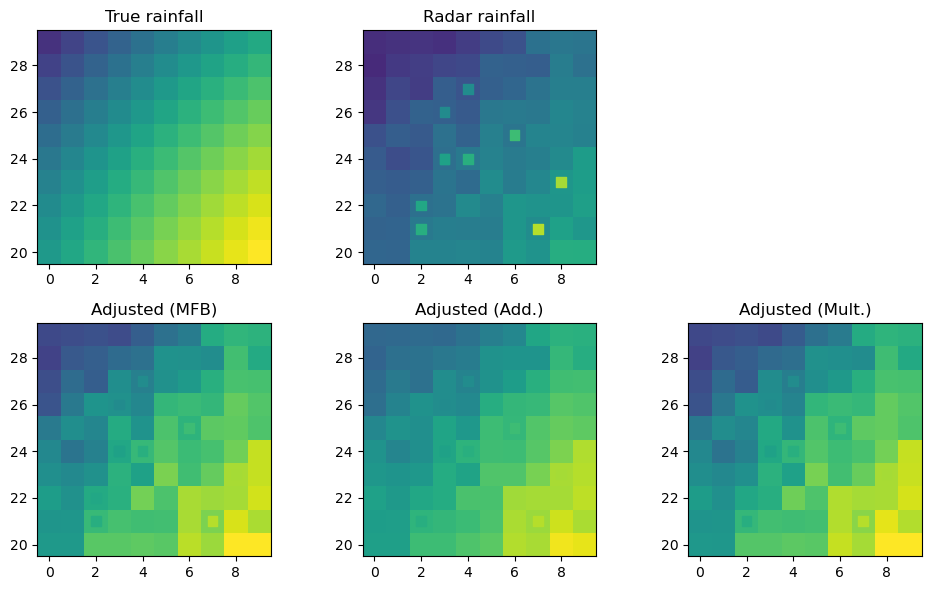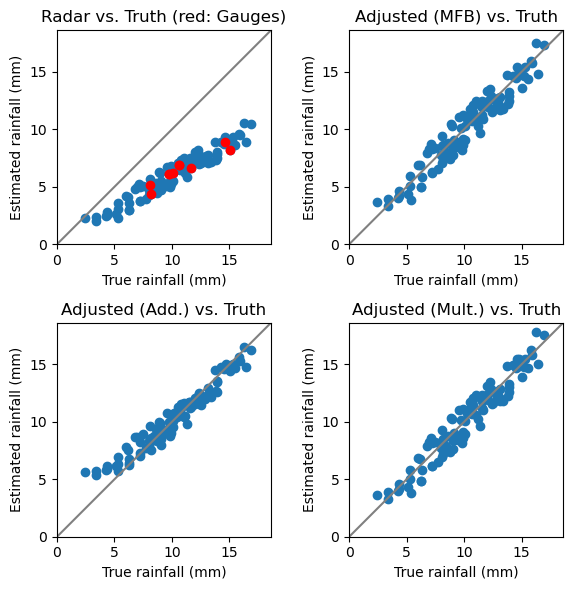## Background¶

$$\omega radlib$$ provides different error models and different spatial interpolation methods to address the adjustment problem. For details, please refer to $$\omega radlib's$$ library reference.

:

import wradlib.adjust as adjust
import numpy as np
import matplotlib.pyplot as pl

try:
get_ipython().run_line_magic("matplotlib inline")
except:
pl.ion()

/home/runner/micromamba-root/envs/wradlib-notebooks/lib/python3.11/site-packages/tqdm/auto.py:22: TqdmWarning: IProgress not found. Please update jupyter and ipywidgets. See https://ipywidgets.readthedocs.io/en/stable/user_install.html
from .autonotebook import tqdm as notebook_tqdm


## Example for the 1-dimensional case¶

Looking at the 1-D (instead of 2-D) case is more illustrative.

### Create synthetic data¶

First, we create synthetic data: - true rainfall, - point observations of the truth, - radar observations of the truth.

The latter is disturbed by some kind of error, e.g. a combination between systemtic and random error.

:

# gage and radar coordinates
obs_coords = np.array([5, 10, 15, 20, 30, 45, 65, 70, 77, 90])

# true rainfall
np.random.seed(1319622840)
truth = np.abs(1.5 + np.sin(0.075 * radar_coords)) + np.random.uniform(
)

errormult = 0.75 + 0.015 * radar_coords

# gage observations are assumed to be perfect
obs = truth[obs_coords]

# add a missing value to observations (just for testing)
obs = np.nan


• additive error, spatially variable (AdjustAdd)

• multiplicative error, spatially variable (AdjustMultiply)

• mixed error, spatially variable (AdjustMixed)

• multiplicative error, spatially uniform (AdjustMFB)

:

# number of neighbours to be used
nnear_raws = 3

)

/home/runner/micromamba-root/envs/wradlib-notebooks/lib/python3.11/site-packages/wradlib/ipol.py:392: RuntimeWarning: divide by zero encountered in divide
weights = 1.0 / self.dists**self.p
a.partition(kth, axis=axis, kind=kind, order=order)


:

# Enlarge all label fonts
font = {"size": 15}
pl.rc("font", **font)

pl.figure(figsize=(10, 5))
pl.plot(
"k-",
linewidth=2.0,
linestyle="dashed",
)
pl.plot(
truth,
"k-",
linewidth=2.0,
label="True rainfall",
)
pl.plot(
obs_coords,
obs,
"o",
markersize=10.0,
markerfacecolor="grey",
label="Gage observation",
)
pl.plot(
)
pl.plot(
)
pl.plot(
"-",
color="blue",
)
pl.xlabel("Distance (km)")
pl.ylabel("Rainfall intensity (mm/h)")
leg = pl.legend(prop={"size": 10})

/tmp/ipykernel_4772/4163946194.py:6: UserWarning: linestyle is redundantly defined by the 'linestyle' keyword argument and the fmt string "k-" (-> linestyle='-'). The keyword argument will take precedence.
pl.plot(### Verification¶

We use the verify module to compare the errors of different adjustment approaches.

Here, we compare the adjustment to the “truth”. In practice, we would carry out a cross validation.

:

# Verification for this example

:

# Helper function for scatter plot
def scatterplot(x, y, title=""):
"""Quick and dirty helper function to produce scatter plots"""
pl.scatter(x, y)
pl.plot([0, 1.2 * maxval], [0, 1.2 * maxval], "-", color="grey")
pl.xlabel("True rainfall (mm)")
pl.ylabel("Estimated rainfall (mm)")
pl.xlim(0, maxval + 0.1 * maxval)
pl.ylim(0, maxval + 0.1 * maxval)
pl.title(title)

:

# Verification reports
maxval = 4.0
# Enlarge all label fonts
font = {"size": 10}
pl.rc("font", **font)
fig = pl.figure(figsize=(14, 8))
ax.text(0.2, maxval, "Nash=%.1f" % rawerror.nash(), fontsize=12)
ax.text(0.2, maxval, "Nash=%.1f" % adderror.nash(), fontsize=12)
ax.text(0.2, maxval, "Nash=%.1f" % multerror.nash(), fontsize=12)
ax.text(0.2, maxval, "Nash=%.1f" % mixerror.nash(), fontsize=12)
scatterplot(mfberror.obs, mfberror.est, title="Mean Field Bias adjustment")
ax.text(0.2, maxval, "Nash=%.1f" % mfberror.nash(), fontsize=12)
pl.tight_layout()## Example for the 2-dimensional case¶

For the 2-D case, we follow the same approach as before:

• create synthetic data: truth, rain gauge observations, radar-based rainfall estimates

• verification

The way these synthetic data are created is totally arbitrary - it’s just to show how the methods are applied.

### Create 2-D synthetic data¶

:

# grid axes
xgrid = np.arange(0, 10)
ygrid = np.arange(20, 30)

# number of observations
num_obs = 10

# create grid
gridshape = len(xgrid), len(ygrid)
grid_coords = util.gridaspoints(ygrid, xgrid)

# Synthetic true rainfall
truth = np.abs(10.0 * np.sin(0.1 * grid_coords).sum(axis=1))

# Creating radar data by perturbing truth with multiplicative and
# YOU CAN EXPERIMENT WITH THE ERROR STRUCTURE
np.random.seed(1319622840)
radar = 0.6 * truth + 1.0 * np.random.uniform(low=-1.0, high=1, size=len(truth))

# indices for creating obs from raw (random placement of gauges)
obs_ix = np.random.uniform(low=0, high=len(grid_coords), size=num_obs).astype("i4")

# creating obs_coordinates
obs_coords = grid_coords[obs_ix]

# creating gauge observations from truth
obs = truth[obs_ix]


:

# Mean Field Bias Adjustment

# Multiplicative Error Model

/home/runner/micromamba-root/envs/wradlib-notebooks/lib/python3.11/site-packages/wradlib/adjust.py:658: FutureWarning: rcond parameter will change to the default of machine precision times max(M, N) where M and N are the input matrix dimensions.
To use the future default and silence this warning we advise to pass rcond=None, to keep using the old, explicitly pass rcond=-1.
slope, _, _, _ = np.linalg.lstsq(x, y)


:

# Helper functions for grid plots
def gridplot(data, title):
"""Quick and dirty helper function to produce a grid plot"""
xplot = np.append(xgrid, xgrid[-1] + 1.0) - 0.5
yplot = np.append(ygrid, ygrid[-1] + 1.0) - 0.5
grd = ax.pcolormesh(xplot, yplot, data.reshape(gridshape), vmin=0, vmax=maxval)
ax.scatter(
obs_coords[:, 0],
obs_coords[:, 1],
c=obs.ravel(),
marker="s",
s=50,
vmin=0,
vmax=maxval,
)
# pl.colorbar(grd, shrink=0.5)
pl.title(title)

:

# Maximum value (used for normalisation of colorscales)

# open figure
fig = pl.figure(figsize=(10, 6))

# True rainfall
gridplot(truth, "True rainfall")

pl.tight_layout():

# Open figure
fig = pl.figure(figsize=(6, 6))

# Scatter plot radar vs. observations